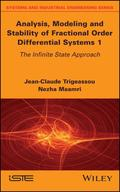John Wiley & Sons Analysis, Modeling and Stability of Fractional Order Differential Systems 1This book introduces an original fractional calculus methodology ('the infinite state approach') whi.. Product #: 978-1-78630-269-4 Regular price: \$142.06 \$142.06 In Stock

# Analysis, Modeling and Stability of Fractional Order Differential Systems 1

## The Infinite State Approach1. Edition August 2019
316 Pages, Hardcover
Wiley & Sons Ltd

ISBN: 978-1-78630-269-4
John Wiley & Sons

### Price: 152,00 €

Price incl. VAT, excl. Shipping

This book introduces an original fractional calculus methodology ('the infinite state approach') which is applied to the modeling of fractional order differential equations (FDEs) and systems (FDSs). Its modeling is based on the frequency distributed fractional integrator, while the resulting model corresponds to an integer order and infinite dimension state space representation. This original modeling allows the theoretical concepts of integer order systems to be generalized to fractional systems, with a particular emphasis on a convolution formulation.

1. The Fractional Integrator.
2. Frequency Approach to the Synthesis of the Fractional Integrator.
3. Comparison of Two Simulation Techniques.
4. Fractional Modeling of the Diffusive Interface.
5. Modeling of Physical Systems with Fractional Models: an Illustrative Example.
6. The Distributed Model of the Fractional Integrator.
7. Modeling of FDEs and FDSs.
8. Fractional Differentiation.
9. Analytical Expressions of FDS Transients.
10. Infinite State and Fractional Differentiation of Functions.
Jean-Claude Trigeassou is Honorary Professor at Bordeaux University, France, and has been associated with the research activities of its IMS-LAPS lab since 2006. His main research interests include the modeling of fractional order systems, based on the infinite state approach.

Nezha Maamri is Associate Professor at Poitiers University, France. Her research activities concern the method of moments, robust control using integer order and fractional order controllers, plus the modeling, initialization and stability of fractional order systems.